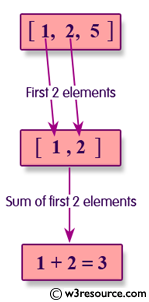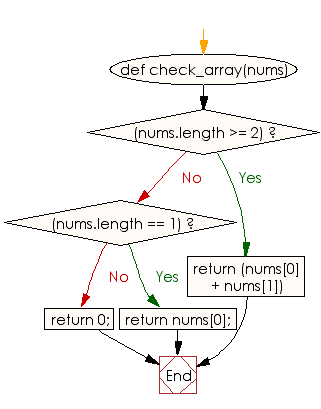﻿ Ruby Array exercises: Compute the sum of the first 2 elements of a given array of integers - w3resource# Ruby Array Exercises: Compute the sum of the first 2 elements of a given array of integers

## Ruby Array: Exercise-14 with Solution

Write a Ruby program to compute the sum of the first 2 elements of a given array of integers. If the array length is less than 2, just sum up the elements that exist, returning 0 if the length of the array is 0.Ruby Code:

``````def check_array(nums)
if(nums.length >= 2)
return (nums + nums)
end
if(nums.length == 1)
return nums;
end
return 0;

end
print check_array([1, 2, 5]),"\n"
print check_array([4, 2, 3]),"\n"
print check_array()
``````

Output:

```3
6
1
```

Flowchart:Ruby Code Editor: